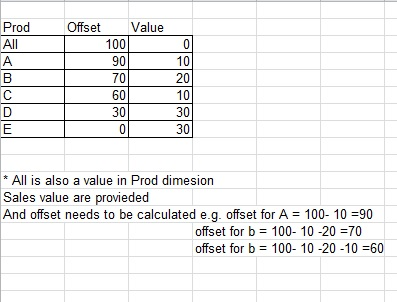# QlikView Layout & Visualizations

Discussion Board for collaboration on QlikView Layout & Visualizations.

Contributor

## Chart help?

Hithis needs to be done using chart expresssions.

thanks for help

Tags (3)
1 Solution

Accepted Solutions
HighlightedMVP

## Re: Chart help?

Try this expression:

=If(Prod = 'All', Sum(TOTAL Value), Sum(TOTAL Value)-RangeSum(Above(Sum(Value), 0, RowNo()-1)))2 Replies
HighlightedMVP

## Re: Chart help?

Try this expression:

=If(Prod = 'All', Sum(TOTAL Value), Sum(TOTAL Value)-RangeSum(Above(Sum(Value), 0, RowNo()-1)))Highlighted
Contributor

## Re: Chart help?

Quite close, it worked.

Thanks